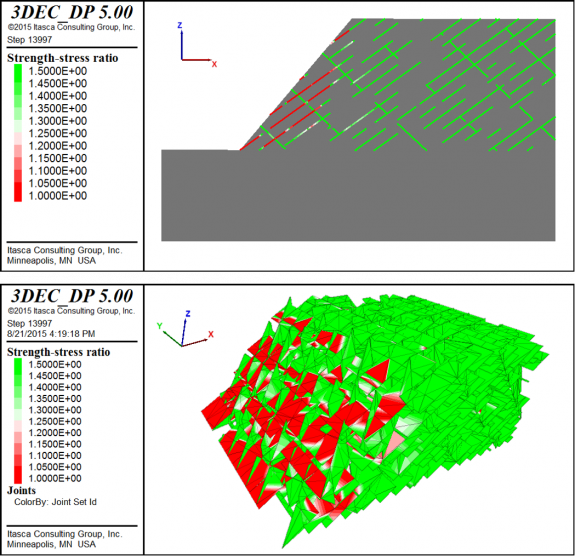## Strength-stress ratio on joints in 3DEC

In 3DEC, it is easy to see if joints have failed by plotting Joints – Slip. This will plot small cubes at each subcontact colored by their state (slipping, slipped in past, or failed in tension). However, it is not so easy to find the joints that are close to failure but have not yet failed. You could run a factor of safety analysis to find the critical joints, but this can be very slow for large 3D models. Another alternative is to plot the strength-stress ratio on each joint.

The strength-stress ratio is the shear strength of the joint divided by the current shear stress, which means large numbers indicate low stress and safe joints. But as the stress approaches the strength, the ratio will approach 1—just like a factor of safety. You could even just solve your model elastically (solve elastic only), and then plot the strength-stress ratio to observe potential problem areas before you even run any more calculation steps!

A FISH function is provided below to enable the plotting of the shear strength-stress ratio for joints. Simply run the function and then plot Joints – Contours – Subcontact Extra. Since the joint contour plots do not have a wireframe (yet), it is helpful to plot Joints on top and turn off the fill so you can get some depth perception in 3D. You could also plot the contours in a reverse green-red color scheme with a modified maximum and minimum (say, 1.0 to 1.5) so that the green areas show as safe and the red areas are the problem areas. An example jointed slope model is shown below.